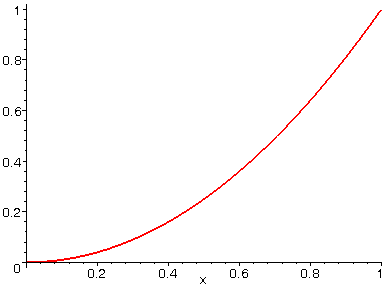RIEMANN SUMS

One of the classic problems that led to the development of calculus was how do we find the area of a region that isn't simple like a rectangle, triangle, or circle? The solution to this problem led to the development of integral calculus, and the the first step in solving this problem involves using rectangles to get an approximate answer. In the animation below, we are interested in how one might approximate the area between the curve y = x^2 and the x-axis over the interval from 0 to 1. This animation illustrates how one might estimate the area by drawing rectangles under the curve and adding up their areas, and how the approximation might improve as the number of rectangles increases. Also, mathematicians refer to an approximation such as the one below as a Riemann sum. Watching this animation is loads of fun. Especially on nights when there's nothing good on TV!

> with(plots): with(student):

> A := seq(leftbox(x^2,x=0..1,i), i=1..50):

> display(A, insequence=true);>